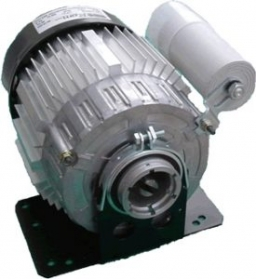# Kilograms 19123

They were to dispatch and transport 35 machine tools of two types with a total weight of 16.54 tons from the plant. The machine of the first type weighed 420 kg. The second was 80 kilograms heavier. How many machines of the first and second kind did they ship?

a =  12
b =  23

### Step-by-step explanation:

a+b=35
16.54=0.420a+(0.420+0.080)b

a+b=35
16.54=0.420·a+(0.420+0.080)·b

a+b = 35
0.42a+0.5b = 16.54

Row 2 - 0.42 · Row 1 → Row 2
a+b = 35
0.08b = 1.84

b = 1.84/0.08 = 23
a = 35-b = 35-23 = 12

a = 12
b = 23

Our linear equations calculator calculates it.Did you find an error or inaccuracy? Feel free to write us. Thank you!

Tips for related online calculators
Do you have a system of equations and looking for calculator system of linear equations?